The read() function in Python, used to read the content of a file. This function is used to read the whole content or first few bytes of content from the file at once.

The syntax to use read() function in Python is:

`fo.read(size)`

where fo indicates to the file object or handler. The size must be given in bytes if you want to read first specified number of bytes (characters) from the file. One byte equals to one character.

Note: The size parameter of read() is optional. That is, if you'll not provide any parameter to the read() function, then the whole content of the file gets returned.

Before proceeding the program, let me create a file say codescracker.txt with some content. Save this file inside the current directory. Here is the snapshot of the current directory, including the opened newly created file.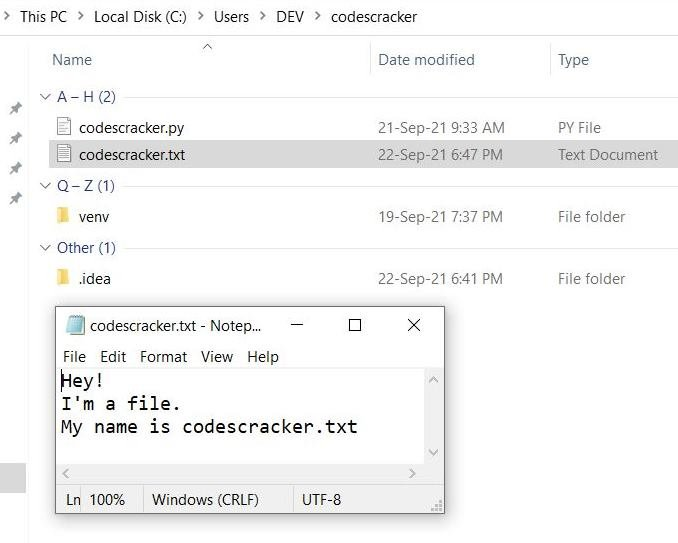Now let's create a program in Python, that uses read() function to read the content of this newly created file:

```fo = open("codescracker.txt", "r")
print(content)```

The output produced by above program will be the content available inside the file codescracker.txt as shown in the snapshot given below, taken when I've executed this program: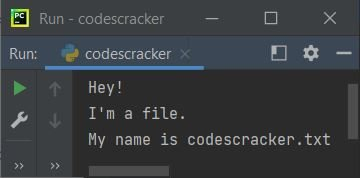In above program, the following two statements:

```content = fo.read()
print(content)```

can also be replaced with single statement as given below:

`print(fo.read())`

Now let's modify the above program, that receives the name of file from user at run-time. This program also handles error raised when the given file does not exists:

```print("Enter the Name of File: ", end="")
filename = input()
try:
fo = open(filename, "r")
print("\n----The content of file \"", filename, "\"----", sep="")
except FileNotFoundError:
print("\nThe file \"", filename, "\" doesn't found.", sep="")```

Here is its sample run with user input codescracker.txt: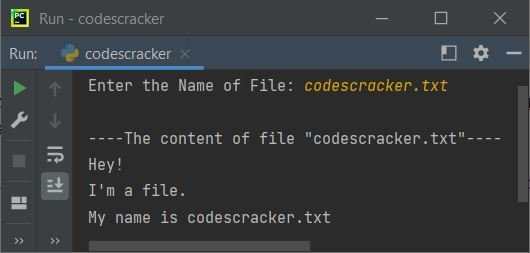And here is another sample run with user input none.txt, a non-existing file: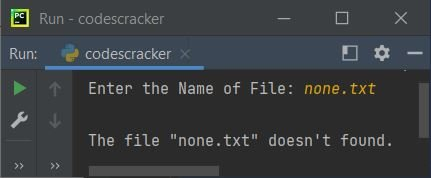Note: The end= and sep=, both parameters are used to change the default behavior of print(). To learn in detail, refer to its separate tutorial.

## Python read() With size Parameter

Now let's create another program, that reads only particular bytes of content from the same file as created earlier. For example, the program given below reads only first 10 bytes from the file:

```fo = open("codescracker.txt", "r")

Here is its sample output:See, the first 10 characters gets returned from the file. Those 10 characters are:

1. H
2. e
3. y
4. !
5. (a newline)
6. I
7. '
8. m
9. (a space)
10. a

Now let me again modify the above program, with the complete package of read() in Python, including handling of invalid inputs:

```print("Enter the Name of File: ", end="")
filename = input()
try:
fo = open(filename, "r")
print("2. Read Only Specified Number of Bytes.")
print("Enter Your Choice (1 or 2): ", end="")
try:
choice = int(input())
if choice==1:
print("\n----The content of file \"", filename, "\"----", sep="")
elif choice==2:
print("\nEnter the Number of Bytes to Read: ", end="")
try:
nob = int(input())
print("\n----The First ", nob, " characters of file \"", filename, "\"----", sep="")
except ValueError:
print("\nInvalid Input!")
except ValueError:
print("\nInvalid Input!")
except FileNotFoundError:
print("\nThe file \"", filename, "\" doesn't found.", sep="")```

The sample run of above program with file name input as same file, and choice as 2 to read specified number of bytes, then 15 as number of bytes to read, is shown in the snapshot given below: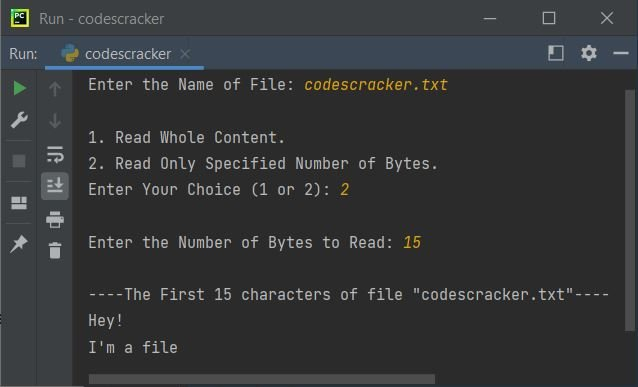Python Online Test

« Previous Function Next Function »